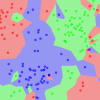# Archive | Code Algorithms From Scratch## Develop k-Nearest Neighbors in Python From Scratch

In this tutorial you are going to learn about the k-Nearest Neighbors algorithm including how it works and how to implement it from scratch in Python (without libraries). A simple but powerful approach for making predictions is to use the most similar historical examples to the new data. This is the principle behind the k-Nearest Neighbors […]## Naive Bayes Classifier From Scratch in Python

In this tutorial you are going to learn about the Naive Bayes algorithm including how it works and how to implement it from scratch in Python (without libraries). We can use probability to make predictions in machine learning. Perhaps the most widely used example is called the Naive Bayes algorithm. Not only is it straightforward […]## What is a Confusion Matrix in Machine Learning

Make the Confusion Matrix Less Confusing. A confusion matrix is a technique for summarizing the performance of a classification algorithm. Classification accuracy alone can be misleading if you have an unequal number of observations in each class or if you have more than two classes in your dataset. Calculating a confusion matrix can give you […]## How to Implement Stacked Generalization (Stacking) From Scratch With Python

Code a Stacking Ensemble From Scratch in Python, Step-by-Step. Ensemble methods are an excellent way to improve predictive performance on your machine learning problems. Stacked Generalization or stacking is an ensemble technique that uses a new model to learn how to best combine the predictions from two or more models trained on your dataset. In […]## How to Implement Random Forest From Scratch in Python

Decision trees can suffer from high variance which makes their results fragile to the specific training data used. Building multiple models from samples of your training data, called bagging, can reduce this variance, but the trees are highly correlated. Random Forest is an extension of bagging that in addition to building trees based on multiple […]## How to Implement Bagging From Scratch With Python

Decision trees are a simple and powerful predictive modeling technique, but they suffer from high-variance. This means that trees can get very different results given different training data. A technique to make decision trees more robust and to achieve better performance is called bootstrap aggregation or bagging for short. In this tutorial, you will discover […]## How To Implement The Decision Tree Algorithm From Scratch In Python

Decision trees are a powerful prediction method and extremely popular. They are popular because the final model is so easy to understand by practitioners and domain experts alike. The final decision tree can explain exactly why a specific prediction was made, making it very attractive for operational use. Decision trees also provide the foundation for […]## How to Code a Neural Network with Backpropagation In Python (from scratch)

The backpropagation algorithm is used in the classical feed-forward artificial neural network. It is the technique still used to train large deep learning networks. In this tutorial, you will discover how to implement the backpropagation algorithm for a neural network from scratch with Python. After completing this tutorial, you will know: How to forward-propagate an […]## How To Implement Learning Vector Quantization (LVQ) From Scratch With Python

A limitation of k-Nearest Neighbors is that you must keep a large database of training examples in order to make predictions. The Learning Vector Quantization algorithm addresses this by learning a much smaller subset of patterns that best represent the training data. In this tutorial, you will discover how to implement the Learning Vector Quantization […]## How To Implement The Perceptron Algorithm From Scratch In Python

The Perceptron algorithm is the simplest type of artificial neural network. It is a model of a single neuron that can be used for two-class classification problems and provides the foundation for later developing much larger networks. In this tutorial, you will discover how to implement the Perceptron algorithm from scratch with Python. After completing […]